Chin. Phys. B, 2020, Vol. 29(10): 100304    DOI: 10.1088/1674-1056/abab72
 General Prev   Next

# Lattice configurations in spin-1 Bose–Einstein condensates with the SU(3) spin–orbit coupling

Ji-Guo Wang(王继国)1,2,†(), Yue-Qing Li(李月晴)1,2, Yu-Fei Dong(董雨菲)1,2
1 Department of Mathematics and Physics, Shijiazhuang TieDao University, Shijiazhuang 050043, China
2 Institute of Applied Physics, Shijiazhuang TieDao University, Shijiazhuang 050043, China
Abstract

We consider the SU(3) spin–orbit coupled spin-1 Bose–Einstein condensates in a two-dimensional harmonic trap. The competition between the SU(3) spin–orbit coupling and the spin-exchange interaction results in a rich variety of lattice configurations. The ground-state phase diagram spanned by the isotropic SU(3) spin–orbit coupling and the spin–spin interaction is presented. Five ground-state phases can be identified on the phase diagram, including the plane wave phase, the stripe phase, the kagome lattice phase, the stripe-honeycomb lattice phase, and the honeycomb hexagonal lattice phase. The system undergoes a sequence of phase transitions from the rectangular lattice phase to the honeycomb hexagonal lattice phase, and to the triangular lattice phase in spin-1 Bose–Einstein condensates with anisotrpic SU(3) spin–orbit coupling.

Keywords:  Bose-Einstein condensates      lattice configurations      SU(3) spin-orbit coupling
Received:  06 April 2020      Revised:  02 July 2020      Published:  05 October 2020
 PACS: 03.75.Lm (Tunneling, Josephson effect, Bose-Einstein condensates in periodic potentials, solitons, vortices, and topological excitations) 03.75.Mn (Multicomponent condensates; spinor condensates) 67.85.Hj (Bose-Einstein condensates in optical potentials) 67.80.K- (Other supersolids)
Corresponding Authors:  Corresponding author. E-mail: wangjiguo@stdu.edu.cn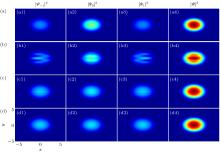Fig. 1.  The ground-state density profiles of ferromagnetic spin-1 BECs with isotropic SU(3) SOC. The columns in every panel from left to right are the densities of the mF = –1 component |Ψ−1|2, the mF = 0 component |Ψ0|2, the mF = 1 component |Ψ1|2, and the total |Ψ|2 = |Ψ−1|2+|Ψ0|2+|Ψ1|2. The SOC strengths in (a)–(d) are γ = 0, 1, 3, and 5, respectively. The spin–spin interaction c2 = −50.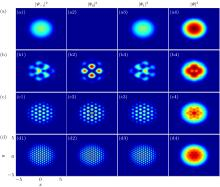Fig. 2.  The ground-state density profiles of antiferromagnetic spin-1 BECs with isotropic SU(3) SOC. The columns in every panel from left to right are the densities of the mF = –1 component |Ψ−1|2, the mF = 0 component |Ψ0|2, the mF = 1 component |Ψ1|2, and the total |Ψ|2 = |Ψ−1|2+|Ψ0|2+|Ψ1|2. The SOC strengths in (a)–(d) are γ = 0, 1, 2, and 4, respectively. The spin–spin interaction c2 = 100.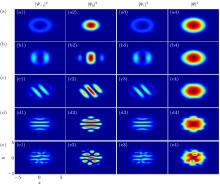Fig. 3.  The ground-state density profiles of antiferromagnetic spin-1 BECs with isotropic SU(3) SOC. The columns in every panel from left to right are the densities of the mF = –1 component |Ψ−1|2, the mF = 0 component |Ψ0|2, the mF = 1 component |Ψ1|2, and the total |Ψ|2 = |Ψ−1|2+|Ψ0|2+|Ψ1|2. The SOC strengths in (a)–(e) are γ = 0.50, 0.75, 1.10, 1.65, and 1.75, respectively. The spin–spin interaction c2 = 2000.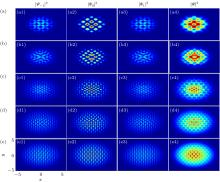Fig. 4.  The ground-state density profiles of antiferromagnetic spin-1 BECs with isotropic SU(3) SOC. The columns in every panel from left to right are the densities of mF = –1 component |Ψ−1|2, mF = 0 component |Ψ0|2, mF = 1 component |Ψ1|2, and the total |Ψ|2 = |Ψ−1|2+|Ψ0|2+|Ψ1|2. The SOC strengths in (a)-(e) are γ = 2, 2.5, 3.5, 4, and 4.4, respectively. The spin–spin interaction c2 = 2000.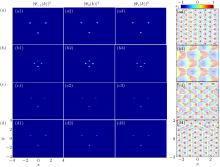Fig. 5.  The parameters of (a)–(d) are the same as those of Figs. 2(d), 4(a), 4(b), and 4(e), respectively. The momentum distributions of (b1)–(d1) the mF = –1 component |Ψ−1(k)|2, (b2)–(d2) the mF = 0 component |Ψ0(k)|2, and (b3)–(d3) the mF = 1 component |Ψ1(k)|2.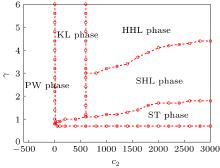Fig. 6.  The ground-state phase diagram spanned by the SU(3) SOC strength γ and the spin–spin interaction strength c2. Five ground-state phases can be identified on the phase diagram, including the PW phase, the ST phase, the KL phase, the SHL phase, and the HHL phase.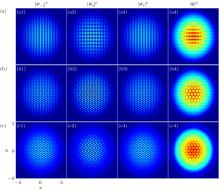Fig. 7.  The ground-state density profiles of spin-1 antiferromagnetic BECs with anisotropic SU(3) SOC. The columns in every panel from left to right are the densities of mF = –1 component |Ψ−1|2, mF = 0 component |Ψ0|2, mF = 1 component |Ψ1|2, and the total |Ψ|2 = |Ψ−1|2+|Ψ0|2+|Ψ1|2. The SOC ratios in (a)–(c) are ζ = 0.90, 0.99 and 1.1, respectively. The spin–spin interaction c2 = 2000 and the SOC strength along the x direction γx = 5.